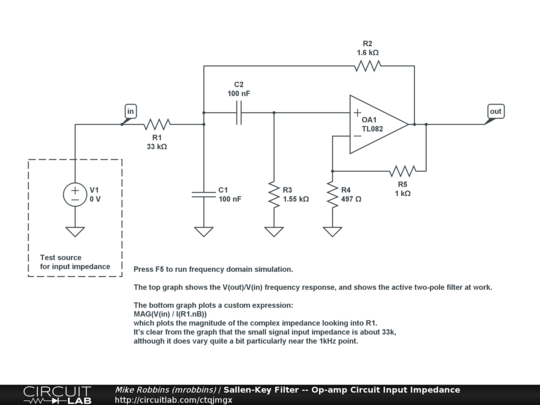# How to compute input impedance?

I use a Sallen-Key filter for a project at university and I need to know its input impedance. Is there a way to compute it theoretically ?

Here is my circuit:• Do you consider the ideal op-amp or the real TL071? May 18, 2012 at 8:35
• @clabacchio : the ideal op-amp May 18, 2012 at 9:59

Yes, this is a standard circuit analysis problem.

Perform the analysis in the frequency domain (R and Xc) and connect a 1A ac current source at the input. Solve for the input voltage as a function of frequency and that expression is the impedance.

I suggest using nodal analysis to perform the analysis.

Assume that the op amp is ideal and so the current into the +/- terminals is zero and the voltage at these terminals are equal.

• I found $$Z_{in}(\omega)=\frac{R_{24}(R_3+R_{23})+j\omega C(R_{23}R_4R_{24}+2R_3R_{23}R_{24}-R_3R_4R_5)+(j\omega)^2C^2R_3R_{23}R_4R_{24}}{R_{24}+j\omega C(2R_{23}R_{24}-R_4R_5)+(j\omega)^2C^2R_{23}R_4R_{24}}$$ which graph is the same (on Matlab) as the one in compumike's post (on CircuitLab) May 17, 2012 at 11:51
• How did you get to it?
– Mu3
May 26, 2017 at 13:53
• @EgorTamarin It's a secret Mar 30, 2019 at 9:42
• I do ot know but the expected result is that the input impedance must be very very high May 24, 2022 at 7:02

Calculating the input impedance by hand is almost certainly what you're supposed to do as the other answers have suggested. I just wanted to show you how to go about getting some numbers out of a circuit simulator so you could check your work (or apply the same concept to a more complicated circuit). Here's your Sallen-Key filter in CircuitLab:And here's the frequency domain simulation showing the input impedance looking into the input:

You can open the circuit and change the parameters, configuration, op-amp model, etc. Just hit F5 and you'll see the V(out)/V(in) Bode plot, as well as the input impedance plot that I've included a screenshot of above. Using custom expressions in the simulator, like MAG(V(in)/I(R1.nB)), allows you to calculate quantities like small signal impedances quite quickly!

Using a test current source, rather than a test voltage source, makes sense for how I'd probably go about solving this on paper. However, for simulation purposes, using a voltage source as the test input allows us to more easily understand the V(out)/V(in) Bode plot at the same time.

@snickers I pretty much just compute the Input Impedance, Zin in my Head.

Well you could solve for it using Ohm's Law and summing node equations, but after you've done it a few times, just do it in your head.

Step 1. Do a DC analysis
Step 2. Do an AC analysis where f is >> fo(BPF)
Step 3. Figure out what happens at f=fo

so here we go.
1. Zin= R1 + R2
2. Zin= R1 (since C5= 0Ω)
3. Zin= open circuit due to cancellation of signals . i.e. no feedback and hence maximum gain.

So if you had one of those nice HP or Anritsu Vector Network Analyzers, you get Zin with a big spike at f0 on a flat line where Zin starts at 35.6kΩ & ends at 33.0kΩ or something close to that...

But I do like the beautiful simulation and graph done above by one of our astute young Engineers.

See it my way? or your way starting with• I get it but what I need is an analytical expression. Anyway useful to estimate it on the go. May 16, 2012 at 20:00

Use the extra element theorem, as explained in Wikipedia. There are multiple paths to the solution with this approach (since any of the components may be made the "extra" one). Choosing C4 as the extra element looks like one of the simpler choices.

In your circuit, the op amp complicates things a bit, but you can write down the currents and voltages on the schematic to compute the various impedances required.

Once you've mastered the extra element theorem, you can then proceed to the generalized N-Extra Element Theorem (NEET, originally developed by S. Sabharwal), which enables you to write down the answer by inspection and a bit of algebra on the schematic:

$$Z_{in}=(R3+R23) \frac{1+s[C5(R3||R23)+C4(R4+(R3||R23)-\frac{(1+R5/R24)}{1+R23/R3}R4)]+s^2C5C4(R3||R23)R4}{1+s[C5R23+C4(R4+R23-(1+R5/R24)R4)]+s^2C5C4R23R4}$$

You can obtain the input impedance of this active circuit using the fast analytical circuits techniques or FACTs. Install a test generator $$\I_T\$$ across the input terminals of your filter. The $$\I_T\$$ current is the stimulus while the voltage $$\V_T\$$ across the source is the response.

First, consider the circuit for $$\s=0\$$: open-circuit all caps. and inspect the circuit below.The input resistance in this condition is simply $$\R_0=R_3+R_{23}\$$.

Now, reduce the excitation to 0 A and open-circuit the current source. Then "look" through the capacitor's connecting terminals to determine the associated time constants in this mode:The first time constant is found by inspection while you need a few equations to get the second one involving $$\C_4\$$. You combine these time constants to form $$\b_1=\tau_5+\tau_4\$$. Then, short $$\C_5\$$ and "look" again through $$\C_4\$$'s terminals to get the new time constant. It's easy, its $$\R_4C_4\$$. You have $$\b_2=\tau_5\tau_{54}\$$. The denominator is equal to $$\D(s)=1+sb_1+s^2b_2\$$.

For the zeroes, consider a response $$\V_T\$$ across the current source equal to zero: we null the response. A zeroed response across a current source is similar to replacing the current source by a short circuit. There we go:The maths are not difficult and you will determine $$\\tau_{5N}\$$, $$\\tau_{4N}\$$ and $$\\tau_{54N}\$$ in the same way as I did in the above lines. The numerator is obtained by combining these time constants together: $$\N(s)=1+s(\tau_{4N}+\tau_{5N})+s^2(\tau_{5N}\tau_{54N})\$$. Finally, the transfer function is $$\Z_{in}(s)=R_0\frac{N(s)}{D(s)}\$$.

I have captured these data in a Mathcad sheet:and the plots are here:A quick SPICE sim tells us this is correct:Some more work can be done to rearrange a bit the transfer function with quality factors in $$\N\$$ and $$\D\$$ but nothing insurmountable. the FACTs are a powerful tool and gets you to the transfer function you want in a few steps that are all verifiable by a simulation.